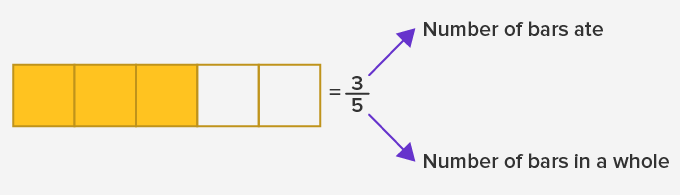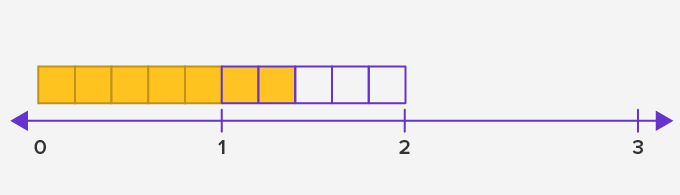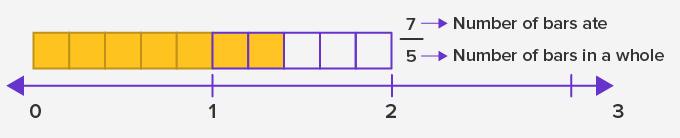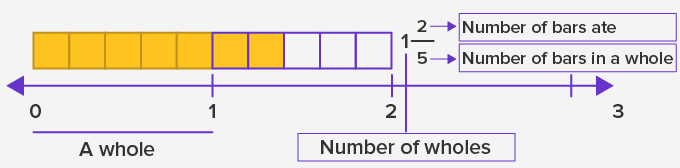# Types Of Fractions - Definition with Examples

The Complete K-5 Math Learning Program Built for Your Child

• 40 Million Kids

Loved by kids and parent worldwide

• 50,000 Schools

Trusted by teachers across schools

• Comprehensive Curriculum

Aligned to Common Core

## Types of Fractions

A fraction represents a part of the whole. In a fraction, the top number is the numerator and the bottom number is the denominator.

For instance:

Kobe has chocolate with 5 bars. If he ate 3 bars of it, then the fraction of the chocolate he ate would beHence, the numerator and the denominator of a fraction inform us about how many parts of the whole there are. The denominator will inform us about the number of equal parts the whole is divided into and the numerator will inform us how many out of the total parts we need to shade.

This leaves us with 2 different cases:

Case 1- When the fraction is less than a wholeThe fractions which are less than a whole are called proper fractions.

Case 2-  When the fraction is more than a wholeThere are two different ways to write a fraction which is more than a whole:2. Mixed FractionExample 1: What is the fraction of the shaded part in the given square?There are 4 parts in the square and 1 part is shaded. The fraction of the shaded part is 1.

## Applications of fractions

Some applications of the fractions are:

(i) Fractions are useful to find out a part of a whole number.

(ii) We use fractions for distribution.

For example: To distribute 6 apples between 2 friends we can find out  12 of  6. We can give 3 apples to each friend.

Types of fractions

There are three types of fractions:

(i) Proper fractions

(ii) Improper fractions

(iii) Mixed fractions

Proper fractions

Proper fractions refer to those fractions where the numerator is smaller than the denominator. A proper fraction is a part of a whole. For example  1,3, 5, 1113

Improper fractions

Improper fractions are those fractions where the numerator is greater than the denominator. They are greater than a whole. For example 75 ,95

Mixed fractions

When we combine a whole number and a proper fraction together, we get a mixed fraction.Fun Fact Egyptians invented fractions as early as 1800 BC. They used fractions for holding the records of the land.

Won Numerous Awards & Honors